# Math Makes Sense: Math 6 Flashcards

Set Details Share
created 9 years ago by stephaniemackay
1,246 views
updated 9 years ago by stephaniemackay
Subjects:
math 6
Page to share:
Embed this setcancel
COPY
code changes based on your size selection
Size:
X

1

IMPROPER FRACTION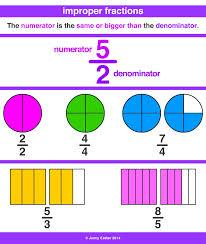A fraction that shows an amount GREATER than one whole. The numerator is GREATER than the denominator. For example ⁷⁄₄ .

2

MIXED NUMBER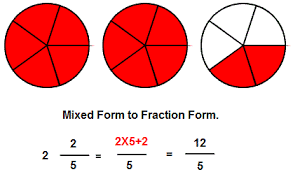A number that has a WHOLE part AND a FRACTION part. For example 1 ⁴⁄₅ .

3

RATIO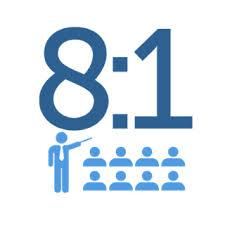A comparison of 2 or more quantities measured with the same unit. For example the number of girls to boys in the class is 6:9.

4

NUMERATOR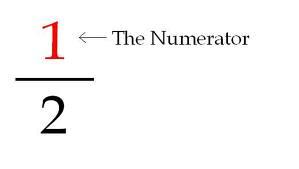The part of a fraction that tells how many equal parts to count. The TOP number in a fraction. For example in ⅔, the numerator is 2.

5

DENOMINATOR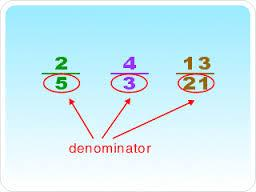The part of a fraction that tells how many equal parts are in one whole. The denominator is the BOTTOM number in a fraction. For example ⅔, the denominator is 3. There are 3 parts in one whole.

6

ACUTE ANGLE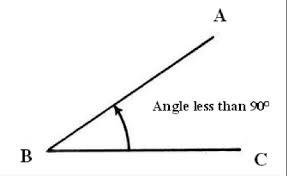An angle that measures less than 90°.

7

OBTUSE ANGLE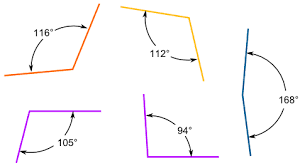An angle that measures more than 90° but less than 180°.

8

REFLEX ANGLE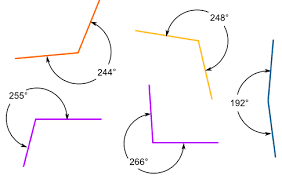An angle that measures more than 180° and less than 360°.

9

ANGLE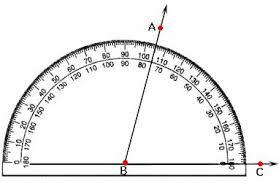Forms when 2 lines meet creating a vertex.

10

RIGHT ANGLE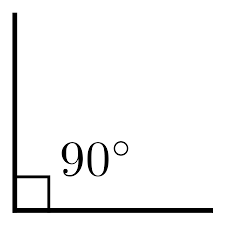An angle that measures exactly 90° and usually indicated by a small square in the angle.

11

STRAIGHT ANGLE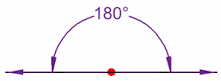An angle that measures exactly 180°. It is a straight line.

12

VARIABLE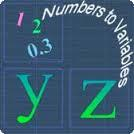A letter that is used to represent a # in an equation or an expression.

3b=12(eq) 4x – 2(ex)

13

CONSTANT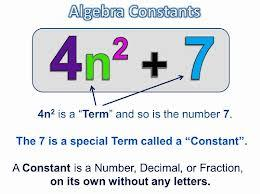In Algebra, a number on its own - a fixed number.

2t + 5

(5 is constantly a pain in the butt and is in a time out and can't play with anyone else)

14

NUMERICAL COEFFICIENT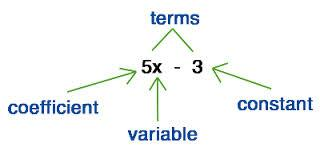A number used to multiply a variable.

4n-2

4 is the term we are describing because it's madly in love with the variable. He's always attached to the variable...ALWAYS!

15

X AXIS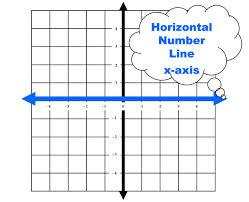The line on a graph that runs horizontally (left-right) through zero.

16

OPERATION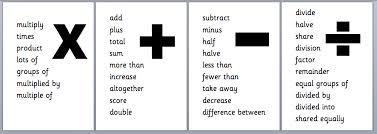A mathematical process.

The most common are add, subtract, multiply and divide (+, -, ×, ÷ ).

17

Y AXIS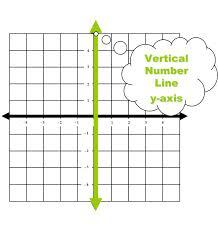The line on a graph that runs vertically (up-down) through zero.

18

ORDERED PAIR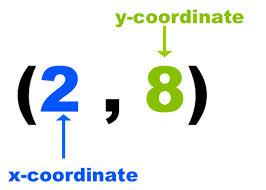Two numbers written in a certain order in parentheses like this: (4,5) or (x,y).
Used to show the position on a graph, where the "x" (horizontal) value is first, and the "y" (vertical) value is second.
Here the point (12,5)

is 12 units to the right, and 5 units up.

19

EQUIVALENT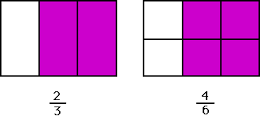Having the same value.

\$1 = 100¢
120 seconds = 2 minutes

5n = 20

20

COMMON MULTIPLE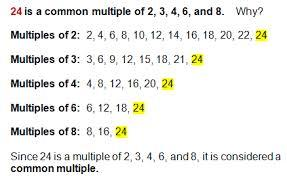A number that is a multiple of 2 or more numbers.

6 is a common multiple of 2 and 3

21

PRIME NUMBER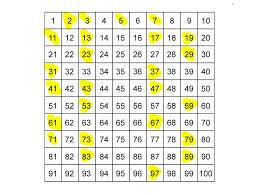A number can be divided evenly only by 1 or itself
2, 3, 5, 7, 9, etc…

22

COMPOSITE NUMBER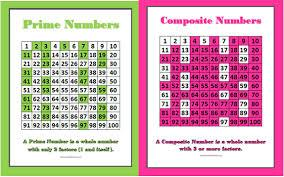A whole number that can be divided evenly by numbers other than 1 or itself. 9=1x9, 3x3

23

COMMON FACTOR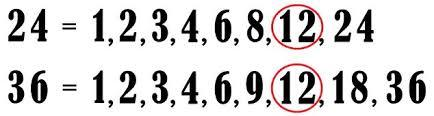A number that is a factor of the given numbers: 3 is a common factor of 15, 9 and 21.

24

ORDER OF OPERATIONS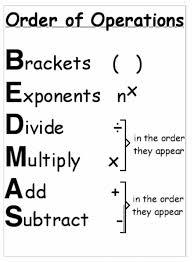The rules of which calculation comes first in an expression. BEDMAS

25

INTEGER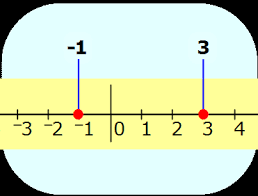A number that is either positive (+) or negative ( - ).

-23, -1, 5, 10, 97. The larger the NEGATIVE number the smaller it is. The larger the POSITIVE number, the bigger the number is.

26

POSITIVE INTEGER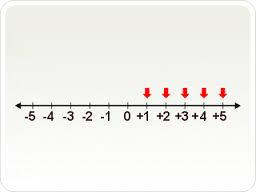Zero is neither negative nor positive.)
5 is positive five and it is found to the RIGHT of ZERO on the number line.

27

NEGATIVE INTEGER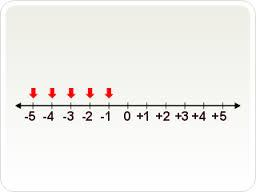Less than zero and written with a minus sign in front of it: -5 is negative five and it is found to the LEFT of ZERO on the number line.

28

ZERO PAIR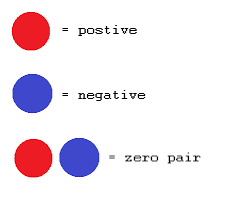A pair of number with a positive and negative sign whose sum is zero. (+,-) For example: +2 and -2 .

29

OPPOSITE INTEGER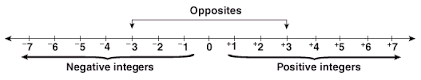For each + integer, there is a - integer, and these integers are called opposites. For example, -3 is the opposite of 3.

30

EXPANDED FORM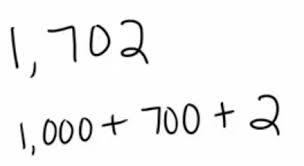Writing a number to show the value of each digit.
It is shown as a sum of each digit multiplied by its matching place value (units, tens, hundreds, etc.)

23 456 = 20000 + 3000 + 400 + 50 +6

31

STANDARD FORM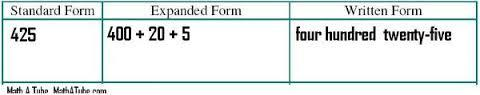A general term meaning "written down in the way most commonly accepted" usually in numbers. For example: 56 347.

32

DEGREE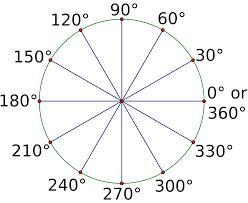A measure for angles. There are 360 degrees in a full rotation (a circle). The symbol for degrees is °. Example: 90 degrees (90°) is a right angle.

33

TRIANGLE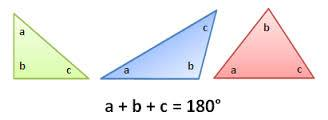The sum of the interior angles of every triangle is equal to 180°.

34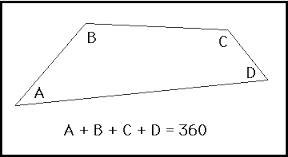The sum of the interior angles of a quadrilateral is equal to 360°.

35

ISOSCELES TRIANGLE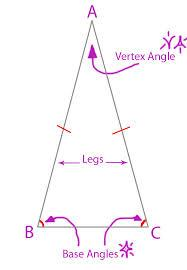A triangle with two = sides, two = angles, and one = line of symmetry. The angles opposite the equal sides are also equal.

36

SCALENE TRIANGLE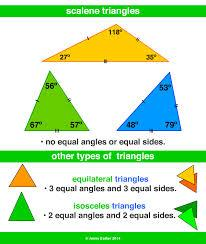A triangle with all s ides and all angles of different lengths and degrees. There is no line of symmetry either.

37

RIGHT TRIANGLE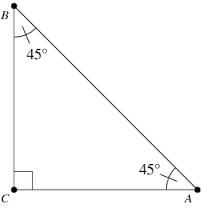A triangle that has a right angle (90°).

38

OBTUSE TRIANGLE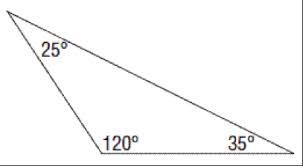A triangle that has ONE angle greater than 90°.

39

PERIMETER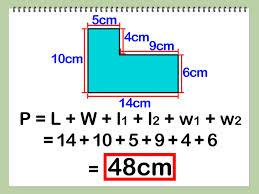The distance around a two-dimensional shape.
Example: it is the total OUTSIDE of a shape (add up every side).

40

IRREGULAR POLYGON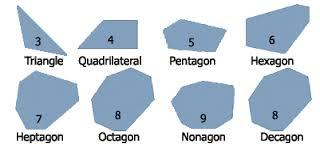A polygon that does not have all sides equal and all angles equal. Even if ONE side is different, it is one of these.

41

REGULAR POLYGON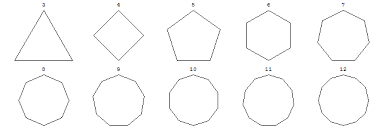A shape where ALL angles and ALL sides are equal.

42

TRANSLATION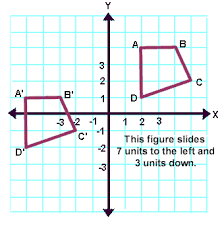Moving a shape, without rotating or flipping it.

"Sliding". The shape still looks exactly the same, the shape is just in a different place.

43

ROTATION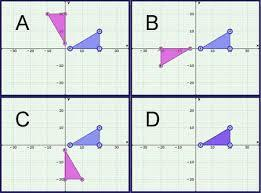A circular movement.
There is a central point that stays fixed and everything else moves around that point in a circle. In other words, stick a NAIL in one corner and spin.

44

REFLECTION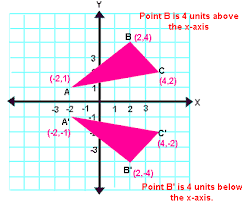An image or shape as it would be seen in a mirror.

45

CONTINUOUS DATA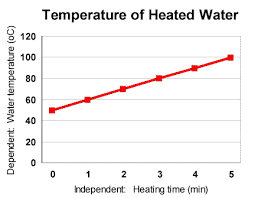Data that can take any value (within a range)
Example: People's heights could be any value (within the range of human heights), not just certain fixed heights.

46

DISCRETE DATA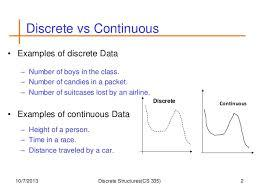Data that can only take certain values.
For example: the number of students in a class (you can't have half a student).

47

PROBABILITY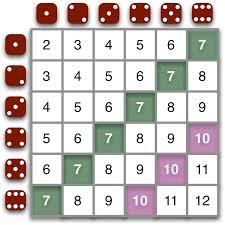Probability is the chance that something will happen - how likely it is that some event will happen.

48

THEORETICAL PROBABILITY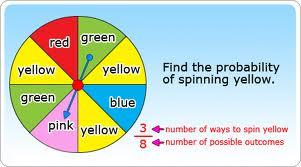The likelihood that an outcome will happen.

In grade 6 terms, this is what is SUPPOSED to happen.

49

EXPERIMENTAL PROBABILITYThe likelihood that something occurs based on the RESULTS of the experiment. In grade 6 terms, this is EXACTLY what happens when you actually DO the experiment.